## Interference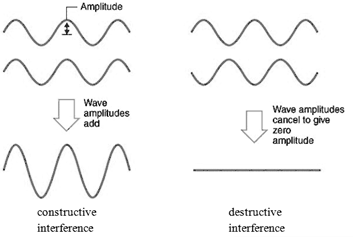All waves interfere. In regions where two light waves overlap, their electric field vectors add.  Light waves with the same polarization can interfere constructively or destructively.  Waves that interfere constructively are in phase, waves that interfere destructively are 180o out of phase.  For the interference to not change with time, the waves have to maintain their phase relationship, they have to be coherent.

How do we make sure two interfering waves have the same polarization?  We split one wave into two waves.  A way to split one wave onto two waves is called division of wave front.  We pass the same wave front through two closely spaced slits.

### The double slit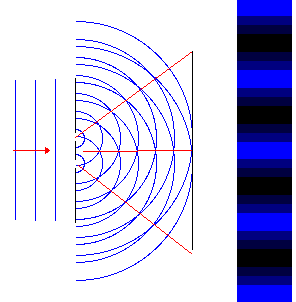If light is incident onto an obstacle which contains two very small slits a distance d apart, then the wavelets emanating from each slit will interfere behind the obstacle.  Waves passing through each slit are diffracted and spread out.  At angles where the single slit diffraction pattern produces nonzero intensity, the waves from the two slits can now constructively or destructively interfere.

If we let the light fall onto a screen behind the obstacle, we will observe a pattern of bright and dark stripes on the screen, in the region where with a single slit we only observe a diffraction maximum.  This pattern of bright and dark lines is known as an interference fringe pattern.  The bright lines indicate constructive interference and the dark lines indicate destructive interference.

The bright fringe in the middle of the diagram on the right is caused by constructive interference of the light from the two slits traveling the same distance to the screen.  It is known as the zero-order fringe.  Crest meets crest and trough meets trough. The dark fringes on either side of the zero-order fringe are caused by destructive interference.  Light from one slit travels a distance that is ½ wavelength longer than the distance traveled by light from the other slit.  Crests meet troughs at these locations.  The dark fringes are followed by the first-order fringes, one on each side of the zero-order fringe.  Light from one slit travels a distance that is one wavelength longer than the distance traveled by light from the other slit to reach these positions.  Crest again meets crest.

Note:  We need single-slit diffraction to observe double-slit interference.  Without the spreading, waves light waves passing through different slits would not meet and therefore could not interfere.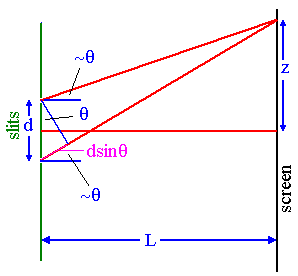The diagram on the right shows the geometry for the fringe pattern.  If light with wavelength λ passes through two slits separated by a distance d, we will observe constructively interference at certain angles a distance L >> d behind the slit.  These angles are found by applying the condition for constructive interference, which is

d sinθ = mλ, m = 0, 1, 2, ... .

The distances from the two slits to the screen differ by an integer number of wavelengths.  Crest meets crest.

The angles at which dark fringes occur can be found be applying the condition for destructive interference, which is

d sinθ = (m+½)λ, m = 0, 1, 2, ... .

The distances from the two slits to the screen differ by an integer number of wavelengths + ½ wavelength.  Crest meets trough.

If this Fraunhofer interference pattern is viewed on a screen a distance L from the slits, then the wavelength can be found from the spacing of the fringes.
We have sinθ = z/(L2 + z2)½ and λ = zd/(m(L2 + z2)½), where z is the distance from the center of the interference pattern to the mth bright line in the pattern.
If L >> z then (L2 + z2)½ ~ L and we can write

λ = zd/(mL).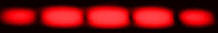Diffraction, and interference are phenomena are observed with all waves.

### Diffraction gratings

We have seen that diffraction patterns can be produced by a single slit or by two slits.  When light encounters an entire array of identical, equally-spaced slits, called a diffraction grating, the bright fringes, which come from constructive interference of the light waves from different slits, are found at the same angles they are found if there are only two slits.  But the pattern is much sharper.
The figure below shows the interference pattern for various numbers of slits. The width of all slits is 50 micrometers and the spacing between all slits is 150 micrometers.  The location of the maxima for two slits is also the location of the maxima for multiple slits.  The single slit diffraction pattern acts as an envelope for the multiple slit interference patterns.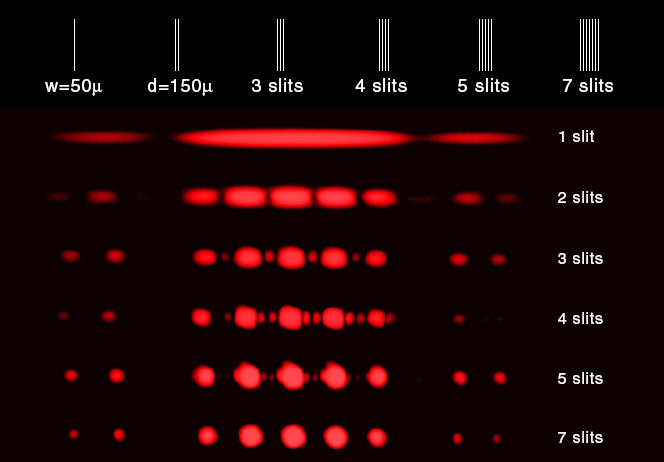Diffraction gratings contain a large number of parallel, closely spaced slits or grooves.  They produce interference maxima at angles θ given by d sinθ = mλ.  Because the spacing between the slits is generally very small, the angles θ are generally quite large.  We cannot use the small angle approximation for relating wavelength and the position of the maxima on a screen for gratings, but have to use

sinθ = z/(L2 + z2)½

Diffraction gratings disperse white light into its component colors because different wavelengths produce bright fringes at different angles.

d sinθ = mλ,
for a given m, bigger wavelength <==> bigger angle

The spectral pattern is repeated on either side of the main pattern.  These repetitions are called "higher order spectra".  There are often many of them, each one fainter than the previous one.  If the distance between slits is d, and the angle to a bright fringe of a particular color is θ, the wavelength of the light can be calculated.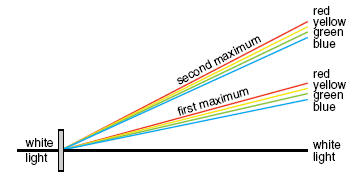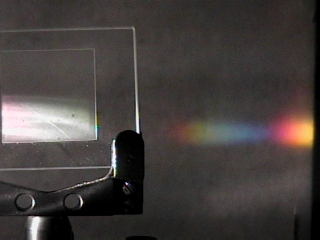Please watch:  Passing Light Through Multiple Apertures - Exploring Wave Motion  (YouTube)

#### Problem:

The first order bright line appears 0.25 cm from the center bright line when a double slit grating is used.  The distance between the slits is 0.5 mm and the screen is 2.7 m from the grating.  Find the wavelength.

Solution:

• Reasoning:
When light with wavelength λ passes through equally spaced slits separated by a distance d, constructively interference occurs at angles θ given by d sinθ = mλ, m = 0, 1, 2, ... .  Here m = 1 and we are asked to solve this equation for λ.
• Details of the calculation:
z = 0.25 cm = 2.5*10-3 m.
L = 2.7 m.
d = 0.5 mm = 5*10-4 m.
L >> z, therefore sinθ ~ z/L and λ = zd/(mL).
λ = (2.5*10-3 m)(5*10-4 m)/(2.7 m).
λ = 4.63*10-7 m = 463 nm.

#### Problem:

A diffraction grating has 420 lines per mm.  The grating is used to observe normally incident light with a wavelength of 440 nm.  The grating is placed 1.3 m from a screen.  Where on the screen will the first order bright line appear?

Solution:

• Reasoning:
When light with wavelength λ passes through equally spaced slits separated by a distance d, constructively interference occurs at angles θ given by d sinθ = mλ, m = 0, 1, 2, ... .  Here m = 1 and we are asked to solve this equation for sinθ and then for z.
• Details of the calculation:
d sinθ = λ.
d = (1/420) mm = 2.38*10-3 mm = 2.38*10-6 m.
λ = 440 nm = 4.40*10-7 m.
L = 1.3 m = distance to the screen.
sinθ = λ/d = 10.65o.
z = L tanθ = 24.5 cm = distance from the central maximum.

#### Problem:

A beam of monochromatic light with a wavelength of 500 nm is directed through an absorber having 5 equally narrow slits separated by 20 μm between adjacent slits. The resulting diffraction pattern is observed on a screen that is perpendicular to the direction of light and 5 m from the slits. The intensity of the central maximum is 1.3 W/m2.
(a)  What are the distances from the central maximum to the first and second principal maxima on the screen?
(b)  What will be the intensity of the central maximum if there are only 4 equally narrow slits (of the same width as in part a), separated by 20 mm between adjacent slits?

Solution:

• Reasoning:
When light with wavelength λ passes through equally spaced slits separated by a distance d, constructively interference occurs at angles θ given by d sinθ = mλ, m = 0, 1, 2, ... .  Here we are asked to solve this equation for sinθ and then for z.  When we have constructive interference, the electric field vectors from all sources point in the same direction and add.  The magnitude of the total field vector is proportional to the number of sources.  The intensity is proportional to the square of the magnitude of the total field vector, and therefore proportional to the square of the number of sources.
• Details of the calculation:
(a)  Constructive interference:
dsinθ = mλ, m = 0, 1, 2, ... .
sinθ = z/(z2 + L2)1/2 ≈ z/L if L >> z.
Here d = 20 mm, λ = 500 nm, L = 5 m.
m = 1: sinθ = 2.5*10-2, sinθ = z/L, z ≈ 12.5 cm.
m = 2: sinθ = 5*10-2, sinθ = z/L, z ≈ 25 cm.

(b)  The central intensity is proportional to the square of the number of sources.
If there are only 4 sources, then the intensity of the central maximum will be
1.3*16/25 W/m2 = 0.832 W/m2.

#### Problem:

A monochromatic wave of wavelength λ illuminates an opaque mask with two slits as shown in the figure. The diffraction pattern is recorded on a screen a distance L from the mask. You may assume that λ << d. D << L.
(a)  What is the distance Λ between adjacent interference fringes observed on the screen?
(b)  What is the width Δx of the central lobe of the interference pattern on the screen?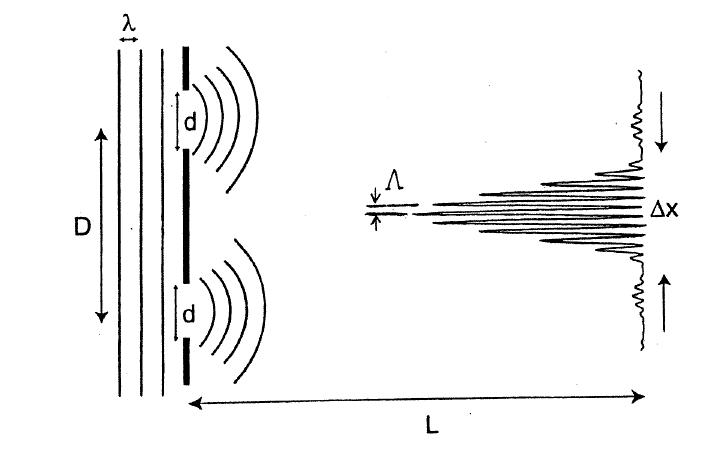Solution:

• Reasoning:
The distance Λ between adjacent interference fringes is the distance between adjacent maxima of the double slit interference pattern.
The width Δx of the central lobe of the interference pattern equals twice the distance from the central maximum to the first minimum of the single slit interference pattern.
• Details of the calculation:
(a)  For the distance between adjacent maxima of the double slit pattern we have Dsinθ = λ.
Here sinθ ≈ θ ≈ Λ/L. So Λ = λL/D.
(b)  For the distance from the central maximum to the first minimum of the single slit interference pattern we have dsinθ = λ.
Here sinθ ≈ θ ≈ Δx /(2L). So Δx = λ2L/d.

Diffraction and interference patterns can be observed when light passes through a set of regularly spaced slits.  For diffraction to produce an observable pattern, the spacing of the slits must be comparable to the wavelength of the radiation.  Visible light has a wavelength range from ~400 nm to ~700 nm.  A typical diffraction grating for visible light with 300 grooves per mm has a slit spacing of (1/300)mm = 3 μm = 3000 nm.  This spacing is 4 to 8 times larger than the wavelengths of visible light and produces an easily observable pattern.

The wavelengths of x-rays lie in the 1 nm to 1 pm range.  A typical diffraction grating will not produce an observable pattern.  But the wavelengths of x-rays are comparable to the spacing of atoms in common crystals, and material with a regularly spaced grid of atoms can diffract x-rays and produce diffraction patterns that can be captured on photographic film.

Interference patterns are only observed if the interfering light from the various sources is coherent, i.e. if the phase difference between the sources is constant.  Splitting the light from a single source into various beams is one way to produce coherent sources.  Light from two different light bulbs is incoherent and will not produce an interference pattern.  Lasers are sources of monochromatic, (single wavelength), coherent light.  Two lasers can maintain a constant phase difference between each other for relatively long time intervals.

#### Module 9, Question 2

• What is the difference between diffraction and interference?
• Compare the formulas for the bright maxima and dark minima in the diffraction and interference patterns.
When you solve problems using these formulas, what do you have to be careful about?

Discuss these questions with your fellow students in the discussion forum!